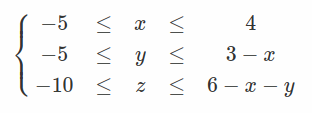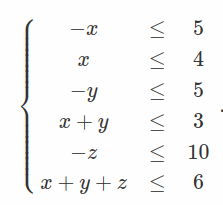# Matrix-vector representation of a system of linear inequalities

Hello,

Consider for example this set of linear inequalities:It is equivalent to:and this can be represented as `A*[x;y;z] <= b` where the matrix `A` is given by the coefficients of the variables at the LHS and the vector `b` is the one made of the bounds at the RHS.

Is it possible and how to get `A` and `b` from given symbolic inequalities `-5 <= x`, `x <= 4`, etc…?

There a ways to do this in JuMP, but they’re not documented: e.g.

1 Like

Thanks! This does not directly gives what I want but it should not be difficult to get what I want from these outputs:

``````using JuMP

model = Model()

@variable(model, x)
@variable(model, y)
@variable(model, z)

@constraint(model, x >= 0)
@constraint(model, x <= 10)
@constraint(model, y >= 0)
@constraint(model, y <= 20-x)
@constraint(model, z >= -5)
@constraint(model, z <= 30-x-y)

undo_relax = relax_integrality(model)

s = JuMP._standard_form_matrix(model)
m, p = size(s.A)
A = s.A[:,1:(p-m)]
``````
``````julia> A
6×3 SparseArrays.SparseMatrixCSC{Float64, Int64} with 9 stored entries:
1.0   ⋅    ⋅
⋅   1.0   ⋅
⋅    ⋅   1.0
1.0   ⋅    ⋅
1.0  1.0   ⋅
1.0  1.0  1.0

julia> s.lower
9-element Vector{Float64}:
-Inf
-Inf
-Inf
0.0
0.0
-5.0
-Inf
-Inf
-Inf

julia> s.upper
9-element Vector{Float64}:
Inf
Inf
Inf
Inf
Inf
Inf
10.0
20.0
30.0
``````

Hmm… I need the possibility to deal with `Rational` coefficients and bounds, and it seems that JuMP only returns some `Float64` stuff.

Thanks to some help I got on SO, I have this feature in Python, with SymPy.

``````def __getAb0(inequalities, symbols, required_type):
# assumptions:
# 1. all inequalities are written with the same relational: < or <= or > or >=
# 2. For each inequality, LHS and RHS are linear in `symbols`
def get_ineq_with_correct_type(i, required_type):
if type(i) != required_type:
i = i.reversed
return i

# extract all inequalities, process them so they are all of the same type and
# terms containing `symbols` are on the LHS.
ineq = []
for i in inequalities:
if isinstance(i, And):
ineq.extend(
[get_ineq_with_correct_type(a, required_type) for a in i.args]
)
else:
ineq.append(get_ineq_with_correct_type(i, required_type))
# at this point, all inequalities should be of the same type.
# rewrite them as expressions: LHS - RHS
equations = [i.lhs - i.rhs for i in ineq]
return linear_eq_to_matrix(equations, symbols)

def getAb(inequalities, symbols):
"""
Get the matrix-vector representation of a set of linear inequalities.

Parameters
----------
inequalities : list
list of symbolic inequalities
symbols : list
list of symbols

Returns
-------
matrix
The matrix of the coefficients of the inequalities.
vector
The vector made of the bounds of the inequalities.

Examples
--------
>>> from pypolyhedralcubature.polyhedralcubature import getAb
>>> from sympy.abc import x, y, z
>>> # linear inequalities
>>> i1 = (x >= -5) & (x <= 4)
>>> i2 = (y >= -5) & (y <= 3 - x)
>>> i3 = (z >= -10) & (z <= 6 - x - y)
>>> # get matrix-vector representation of these inequalities
>>> A, b = getAb([i1, i2, i3], [x, y, z])

"""
A, b = __getAb0(inequalities, symbols, LessThan)
return np.array(A), np.array(b)[:, 0]
``````

No way to do something like that in Julia?Perhaps it is time to make this a public function. People seem to keep wanting it

1 Like

Thanks. I’m aware of SymPy.jl but I have not been able to install it (on Windows). I don’t want to include a dependency with a potential difficulty in installing it.